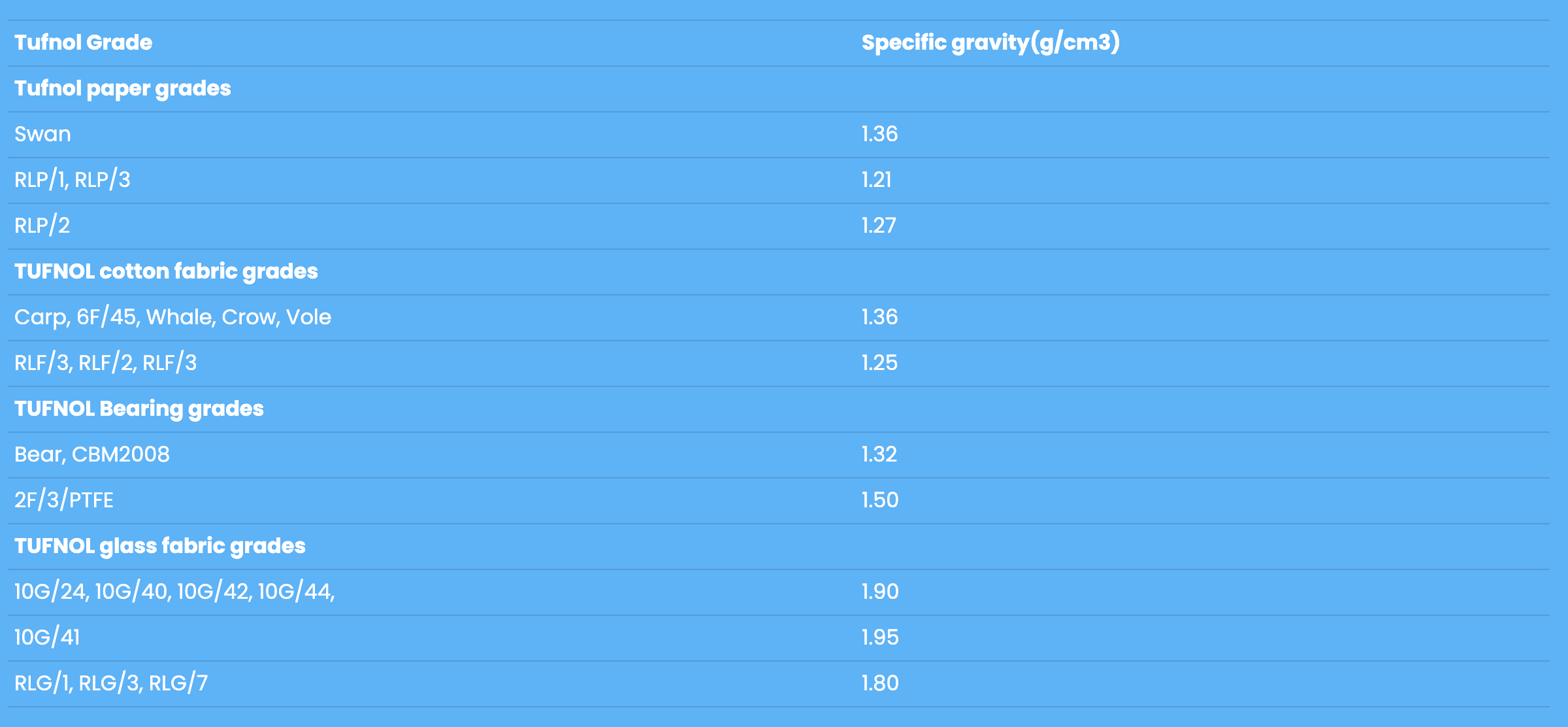# Weights of Tufnol Laminated rods and tubes

Calculate the approximate weight of Tufnol rods as follows:

Calculate weight in kg:

D = diameter in mm

L = length in mm

G = specific gravity for grade (see above)

Weight in kg = 0.007854 x D2 x L x G

Calculate the approximate weight of Tufnol tubes as follows:

Calculate weight in kg.

D = Outside diameter in mm

d = inside diameter in mm

L = length in mm

G = specific gravity for grade (see above)

Weight in kg = 0.007854 x (D2 – d2) x L x GSwan 1.36
RLP/1, RLP/3 1.21
RLP/2 1.27